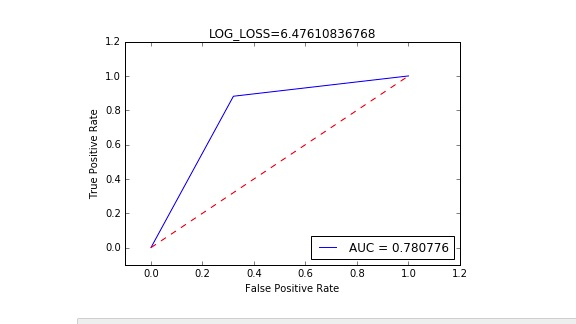# Two issues with Binary Classification

Hi,
I am trying to write a fully working LR example, and I encountered two different issues.
The NN is very simple, with a `torch.nn.Linear` with two targets and as output and a `torch.nn.CrossEntropyLoss()` as the loss function.

https://github.com/QuantScientist/Deep-Learning-Boot-Camp/blob/master/day%2002%20PyTORCH%20and%20PyCUDA/PyTorch/17%20%20PyTorch%20Logistic%20Regression.ipynb

1. The first issue is related to ROC_AUC, during training, which seems to be skwed instead of beeing curve-linear. This happens probably because of the way I am calculating the probabilities but I cant seem to get my hands on where is the error.2. The second issue is during testing, with the dimentions of the results of running the inference, and the dimentions of the actual expected target (e.g. 0 or 1) . Cant seem to be able to fix this, see the picture below.

Many thanks,

EDIT:
First issue resolved by:

1. Changing out dimension to 1 and returning `return F.sigmoid(x)`
2. Changing the loss function to `torch.nn.BCELoss()`
3. During training use `prediction = (net(X).data).float() # probabilities`